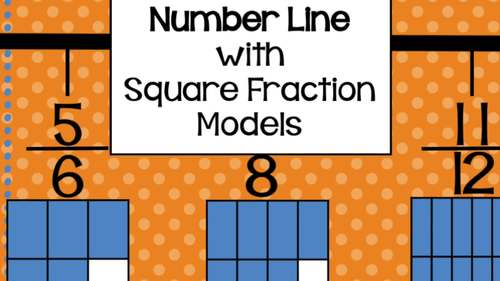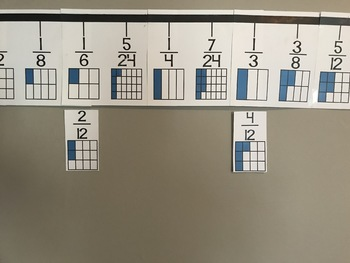# Fraction Number Line with Square Fraction Models for Classroom WallSubject
Resource Type
File Type
PDF (8 MB|53 pages)
Standards
\$5.29
• Product Description
• Standards

This fraction number line will serve as a classroom reference tool, providing students with a foundation of fractions. While the number line hangs in your classroom, fraction equivalence and the relationship between the fractional numbers and their visual representations can be explored.

• On the number line students will see a fractional representation of halves, thirds, fourths, sixths, eighths, twelfths, and twenty-fourths.
• The number line counts with increments of 24ths to make the increments on the number line even. The 24ths can be covered up with the provided blank cards if you don't want to expose your students to these yet.
• Cards are provided to make the fractions equivalent. For example, if you want to show students how 6/12 is the same as ½, you will be able to do so with the provided cards.

• Print this number line and word cards onto card stock. I recommend laminating the number line as well especially if you plan on sticking the equivalence cards to the number line.
• When hung, the number line will be approximately 10 feet long. Because this item will be printed on 8.5 x 11 inch sheets of paper, assembly will be required to attach each page. This will take 15-30 minutes to cut and assemble with tape. I recommend laminating as well.

If you like this product, you may also like Fill in Fraction Number Charts, which count by ½, ⅓.¼, 1/5, 1/6, ⅛, 1/10, 1/12, and 1/100.

You can see other products of mine by visiting my Teachers Pay Teachers store at Ms. K.

Compare two fractions with different numerators and different denominators, e.g., by creating common denominators or numerators, or by comparing to a benchmark fraction such as 1/2. Recognize that comparisons are valid only when the two fractions refer to the same whole. Record the results of comparisons with symbols >, =, or <, and justify the conclusions, e.g., by using a visual fraction model.
Explain why a fraction 𝘢/𝘣 is equivalent to a fraction (𝘯 × 𝘢)/(𝘯 × 𝘣) by using visual fraction models, with attention to how the number and size of the parts differ even though the two fractions themselves are the same size. Use this principle to recognize and generate equivalent fractions.
Understand two fractions as equivalent (equal) if they are the same size, or the same point on a number line.
Explain equivalence of fractions in special cases, and compare fractions by reasoning about their size.
Represent a fraction 𝘢/𝘣 on a number line diagram by marking off 𝘢 lengths 1/𝘣 from 0. Recognize that the resulting interval has size 𝘢/𝘣 and that its endpoint locates the number 𝘢/𝘣 on the number line.
Total Pages
53 pages